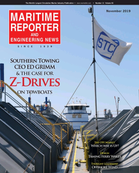# Marine Gears Projects Good Market

M a r i n e Gears — consisting of M a r i n e G e a r s Inc., Haley Clutch a n d C o u p l i n g Corp. a n d M a r i n e G e a r s Intl. — is d e d i c a t e d to s e r v i n g the p r o p u l s i o n needs of the m a r i t i me industry, a n d it's p r i m a r y target is the w o r k b o a t market. M a r i n e Gears has noted a l a r g e increase in the number of r e b u i l d s a n d repowers of existing vessels w i t h i n t h e last decade. It expects this t r e n d t o c o n t i n u e in the near term, w i t h n e w b u i l d numbers holdi n g steady as w e l l . Over the next five to 1 0 years, the c o m p a n y looks f o r the industry as a w h o l e to b e g i n to increase n e w b u i l d i n g projects at a steady pace, a p r o j e c t i on based p r i m a r i l y o n the a g e of the current fleet. To meet customer demands, the comp a n y offers a n a r r a y of new, r e - m a n u f a c t u r e d a n d re-built products. New marine p r o p u l s i o n g e a r s i n c l u d e Pneumatic Clutch G e a r s , vertical o r h o r i z o n t a l offset, in either the FMC series (Flywheel M o u n t e d Clutch) or the G M C series ( G e a r M o u n t e d Clutch).

It also offers H y d r a u l i c Clutch G e a r s , in either the h y d r a u l i c reversing vertical (HRV); h y d r a u l i c reversing h o r i z o n t a l (HRH); o r the reversing in-line (Rl) types.

The Haley HRC Torsional C o u p l i n g is d e s i g n e d to help smooth out the h a m m e r i ng effect i n d i c a t i v e of all m a r i n e p r o p u l s i o n engines, to protect the g e a r elements and b e a r i n g s . They a l s o reduce t o r s i o n a l v i b r a t i o n s t h r o u g h o u t the entire p r o p u l s i o n packa g e a n d p r o v i d e a d a m p e n i n g effect f o r v i b r a t i o n a n d noise transmitted to the vessel f r om the m a i n engines. M a r i n e G e a r s offers m a n y a d d i t i o n a l products, t o o numerous to detail. W h i l e the company's current p r o d u c t arsenal is impressive in b o t h size a nd diversity, it c o n t i n u a l l y evaluates a n d updates to keep up w i t h c h a n g i n g a n d p e n d i ng customer needs a n d legislation. For e x a m p l e , the c o m p a n y has seen e n g i n e manufacturers c h a n g e designs over the past f ew years, substantially i n c r e a s i n g horsepower r a t i n g s on their m a r i n e diesel engines based on customer requirement a n d possible future regulations.